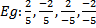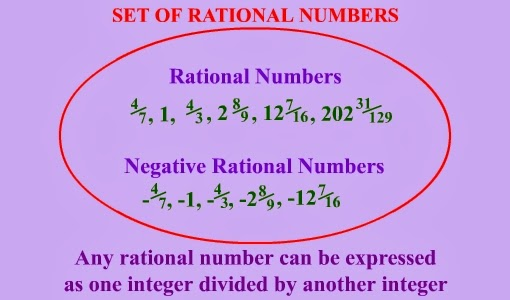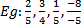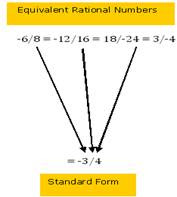## 19 January 2014

All numbers, including whole numbers, integers, fractions and decimal numbers, can be written in the numerator-denominator form.  A rational number is a number that can be written in the form p/q, where p and q are integers and q ≠ 0..The denominator of a rational number can never be zero.  A rational number is positive if its numerator and denominator are both either positive integers or negative integers..  If either the numerator or the denominator of a rational number is a negative integer, then the rational number is called a negative rational number.

The rational number zero is neither negative nor positive.

On the number line:
• Positive rational numbers are represented to the right of 0.
• Negative rational numbers are represented to the left of 0.
By multiplying or dividing both the numerator and the denominator of a rational number by the same non-zero integer, we can get another rational number that is equivalent to the given rational number.
A rational number is said to be in its standard form if its numerator and denominator have no common factor other than 1, and its denominator is a positive integer.To reduce a rational number to its standard form, divide its numerator and denominator by their Highest Common Factor (HCF).  To find the standard form of a rational number with a negative integer as the denominator, divide its numerator and denominator by their HCF with a minus sign.

 With our free PDF notes you can get success in any competitive or entrance exams like CTET,  KVS, NET, CAT, MAT, CMAT, SSC, B.ED, IBPS Recruitment, IAS, CSAT, State Civil Services Exams, UPTET, PSTET, HTET & many more. It also provides NCERT solutions, CBSE, NTSE, Olympiad study material, Indian General Knowledge, English, Hindi, Mathematics, Current affairs, Science, S.ST, model test papers, important Questions and Answers asked in CBSE examinations.